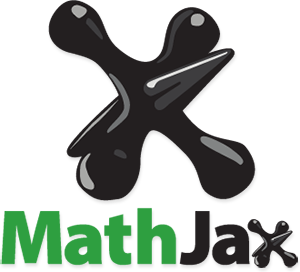# MathJax with Jekyll

##### Posted on February 16, 2014

One of the rewards of switching my website to Jekyll is the ability to support MathJax, which means I can write LaTeX-like equations that get nicely displayed in a web browser, like this one $$\sqrt{\frac{n!}{k!(n-k)!}}$$ or this one $$x^2 + y^2 = r^2$$.### What’s MathJax?

If you check MathJax website (www.mathjax.org) you’ll see that it is an open source JavaScript display engine for mathematics that works in all browsers.

### How to implement MathJax with Jekyll

I followed the instructions described by Dason Kurkiewicz for using Jekyll and Mathjax.

Here are some important details. I had to modify the Ruby library for Markdown in my _config.yml file. Now I’m using redcarpet so the corresponding line in the configuration file is: markdown: redcarpet

To load the MathJax javascript, I added the following lines in my layout page.html (located in my folder _layouts)

Of course you can choose a different file location in your jekyll layouts.

### A Couple of Examples

Here’s a short list of examples. To know more about the details behind MathJax, you can always checked the provided documentation available at http://docs.mathjax.org/en/latest/

I’m assuming you are familiar with LaTeX. However, you should know that MathJax does not have the exactly same behavior as LaTeX. By default, the tex2jax preprocessor defines the LaTeX math delimiters, which are \$$...\$$ for in-line math, and \$...\$ for displayed equations. It also defines the TeX delimiters $$...$$ for displayed equations, but it does not define $...$ as in-line math delimiters. Fortunately, you can change these predefined specifications if you want to do so.

Let’s try a first example. Here’s a dummy equation:

How do you write such expression? Very simple: using double dollar signs

To display inline math use \$$... \$$ like this \$$sin(x^2) \$$ which gets rendered as $$sin(x^2)$$

Here’s another example using type \mathsf

which gets displayed as

Or even better:

is displayed as

$\mathbf{X} = \mathbf{Z} \mathbf{P^\mathsf{T}}$

If you want to use subscripts like this $$\mathbf{X}_{n,p}$$ you need to scape the underscores with a backslash like so \mathbf{X}\_{n,p}:

will be displayed as

$\mathbf{X}_{n,p} = \mathbf{A}_{n,k} \mathbf{B}_{k,p}$

Published in categories opinion  Tagged with resources  jekyll